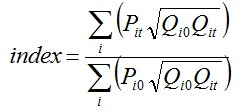# Different ways of measuring the Consumer Price Index (CPI)

Economics, Economic / Financial Data

The consumer price index (CPI) is used as an estimate of the general price level of an economy.

The percentage change in the CPI is used as an estimate of the rate of inflation.

CPI data is gathered by sampling prices and using a ‘basket’ of goods as weights.  This basket would be renewed every few years.  In some countries CPI figures are released monthly, while in other countries they are released quarterly.

The following sections describe some of the different methods for calculating CPI.  These indices are typically multiplied by 1000 so that the base period index would equal 1000.

## Laspeyres Index

Published CPI figures for most countries are usually described as having been calculated using a Laspeyres index, but the actual calculation could differ due to the base period price and quantity / expenditure data being recorded at slightly different times.  The conventional methods are more accurately described as a Lowe index (explained later) that either conforms or closely conforms to a Laspeyres index.

CPI (Laspeyres) formula: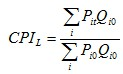Where: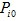is the price of item i at time 0 (the base period)is the price of item i at time t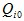is the quantity consumed of item i at time 0

An alternative formula for the Laspeyres index is as follows: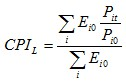Where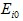is the level of expenditure of item i at time 0.

An advantage of the Laspeyres index is that it is relatively easy to get timely figures.  If you already have the prices and weights for the base period, you would only need prices for the period you want to investigate.  For most other indices, you would need both prices and weights to be up-to-date for that period.

A disadvantage of the Laspeyres index, is that it can suffer from item substitution bias (explained later).

## Paasche Index

The CPI calculated via a Paasche index, helps give an idea of what today’s ‘basket’ would have cost at yesterday’s prices.

CPI (Paasche) formula: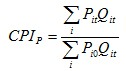Where:is the price of item i at time 0 (the base period)is the price of item i at time t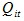is the quantity consumed of item i at time t

An alternative formula for the Paasche index is as follows: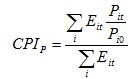Whereis the level of expenditure of item i at time t.

## Fisher Index

The Fisher index is calculated by taking the geometric mean of the Laspeyres and Paasche indices: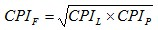The Fisher index helps overcome the problem of item substitution bias.

### Item Substitution Bias

The law of demand states that if the price of a good increases, the quantity demanded of that good decreases, and vice versa (with all other variables remaining the same).

Let’s say the price increases for a good with a high weighting and the price remains the same for a good with a low weighting.  If consumers respond to the price increase of the first good, by substituting it with the second, the overall inflation estimate would be overstated if a Laspeyres index is used.  This is an example of item substitution bias.

The Laspeyres method tends to over-estimate the general price level, while the Paasche method tends to under-estimate it.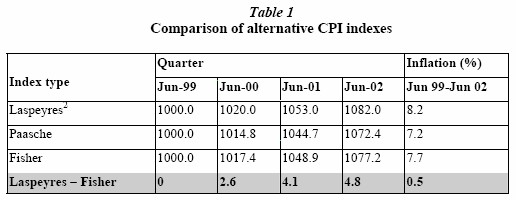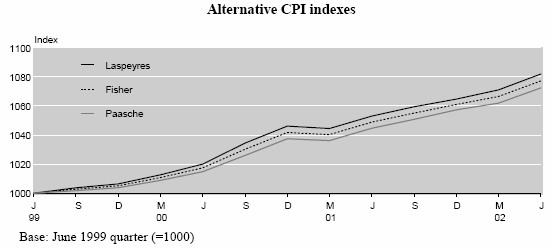The above table and graph are from:
OECD Seminar 2005, Inflation Measures: too high – too low – internationally comparable?  Measurement Issues in the New Zealand Consumers Price Index.

## Lowe Index

The Lowe index has a relatively generalised formula, where the quantities / expenditure weights could potentially have a different reference period to the base or latest periods.  Laspeyres and Paasche indices are special cases of the Lowe index.  The reference period for the weights, could be before the base period.

CPI (Lowe) formula: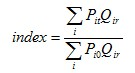Where:is the price of item i at time 0 (the base period)is the price of item i at time t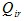is the quantity consumed of item i at time r

An alternative formula for the Lowe index is as follows: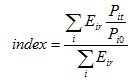Where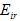is the level of expenditure of item i at time r.

## Törnqvist Index

Like the Fisher index, the Törnqvist index can help overcome item substitution bias.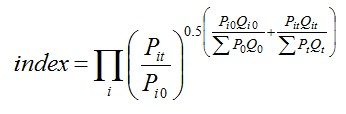OR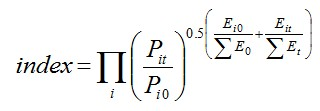## Walsh Index

Like the Fisher index, the Walsh index can help overcome item substitution bias.